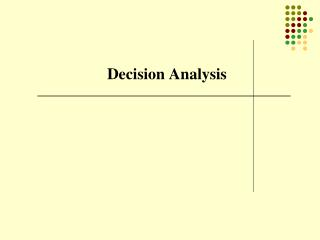Download PresentationDecision Analysis

# Decision Analysis

Download Presentation## Decision Analysis

- - - - - - - - - - - - - - - - - - - - - - - - - - - E N D - - - - - - - - - - - - - - - - - - - - - - - - - - -
##### Presentation Transcript

1. Decision Analysis

2. Basic Terms • Decision Alternatives (eg. Production quantities) • States of Nature (eg. Condition of economy) • Payoffs (\$ outcome of a choice assuming a state of nature) • Criteria (eg. Expected Value)

3. What kinds of problems? • Alternatives known • States of Nature and their probabilities are known. • Payoffscomputableunder different possible scenarios

4. Decision Environments Ignorance – Probabilities of the states of nature are unknown, hence assumed equal Risk / Uncertainty – Probabilities of states of nature are known Certainty – It is known with certainty which state of nature will occur. Trivial problem.

5. Example – Decisions under Ignorance Assume the following payoffs in \$ thousand for 3 alternatives – building 100, 200, or 400 condos. The payoffs depend on how many are sold, which depends on the economy. Three scenarios are considered - a Poor, Average, or Good economy at the time the condos are completed.

6. Maximax - Risk Seeking Behavior What would a risk seeker decide to do? Maximize payoff without regard for risk. In other words, use the MAXIMAX criterion. Find maximum payoff for each alternative, then the maximum of those. The best alternative under this criterion is A3, with a potential payoff of 1200.

7. Maximin – Risk Averse Behavior What would a risk averse person decide to do? Make the best of the worst case scenarios. In other words, use the MAXIMIN criterion. Find minimum payoff for each alternative, then the maximum of those. The best alternative under this criterion is A1, with a worst case scenario of 300, which is better than other worst cases.

8. LaPlace – the Average What would a person somewhere in the middle of the two extremes choose to do? Take an average of the possible payoffs. In other words, use the LaPlace criterion (named after mathematician Pierre LaPlace). Find the average payoff for each alternative, then the maximum of those. The best alternative under this criterion is A2, with an average payoff of 400, which is better than the other two averages.

9. Minimax Regret – Lost Opportunity What would a person choose who wanted to minimize the worst mistake possible? For each state of nature, find the maximum payoff, and subtract each of the payoffs from it to compute the lost opportunities (regrets). Then find maximum values for each alternative, and the minimum of those. The best alternative under this criterion is A2, with a maximum regret of 500, which is better than the other two maximum regrets.

10. Example – Decisions under Risk Assume now that the probabilities of the states of nature are known, as shown below.

11. Expected Values When probabilities are known, compute a weighed average of payoffs, called the Expected Value, for each alternative and choose the maximum value. The best alternative under this criterion is A2, with a maximum EV of 400, which is better than the other two EVs.

12. Expected Opportunity Loss (EOL) Compute the weighted average of the opportunity losses for each alternative to yield the EOL. The best alternative under this criterion is A2, with a minimum EOL of 170, which is better than the other two EOLs. Note that EV + EOL is constant for each alternative! Why?

13. EVUPI: EV with Perfect Information If you knew everytime with certainty which state of nature was going to occur, you would choose the best alternative for each state of nature every time. Thus the EV would be the weighted average of the best value for each state. Take the best times the probability, and add them all. 300*0.3 = 90 600*0.6 = 360 1200*0.1 = 120 _____________ Sum = 570 Thus EVUPI = 570

14. EVPI: Value of Perfect Information Since EVUPI is 570, and you could have made 400 in the long run (best EV without perfect information), the value of this additional information is 570 - 400 = 170. Thus, EVPI = EVUPI – Evmax = EOLmin If someone offered you perfect information about which state of nature was going to occur, how much is that information worth to you in this decision context?

15. Decision Tree 300 0.3 340 0.6 350 0.1 400 A1 -100 0.3 A2 0.6 600 A2 400 400 0.1 700 A3 0.3 -1000 0.6 -200 -300 0.1 1200

16. Sequential Decisions • Would you hire a consultant (or a psychic) to get more info about states of nature? • How would additional info cause you to revise your probabilities of states of nature occuring? • Draw a new tree depicting the complete problem.

17. Consultant’s Track Record

18. Probabilities • P(F/S1) = 0.2 P(U/S1) = 0.8 • P(F/S2) = 0.6 P(U/S2) = 0.4 • P(F/S3) = 0.7 P(U/S3) = 0.3 • F= Favorable U=Unfavorable

19. Joint Probabilities

20. Posterior Probabilities • P(S1/F) = 0.06/0.49 = 0.122 • P(S2/F) = 0.36/0.49 = 0.735 • P(S3/F) = 0.07/0.49 = 0.143 • P(S1/U) = 0.24/0.51 = 0.47 • P(S2/U) = 0.24/0.51 = 0.47 • P(S3/U) = 0.03/0.51 = 0.06

21. Solution • Solve the decision tree using the posterior probabilities just computed.